Polygons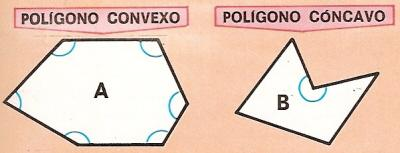1. Convex and concave polygon

A polygon is a closed plane figure made up of several line segments that are joined together.

In the picture, there are two polygons: A and B.

Every angle of the polygon A is less than or equal to 180░. It is called a convex polygon.

The polygon B has an angle that is greater than 180░. It is called concave polygon.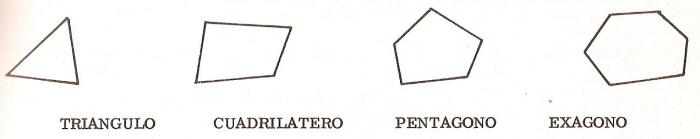2. Types of polygons based on number of sides

We have the following polygons: the triangle (three-sided polygon), the quadrilateral (four-sided polygon), the pentagon (five-sided polygon), the hexagon (six-sided polygon), heptagon (seven-sided polygon), the octagon (eight-sided polygon), the nonagon (nine-sided polygon) and the decagon (ten-sided polygon).The polygon A is... The polygon B is... The polygon C is... The polygon D is... The polygon E is...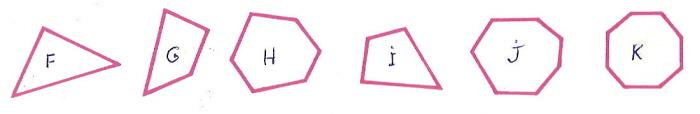3. Irregular Polygons

Note that the polygons F to K do not have all sides equal and all angles equal. These polygons are called irregular.

 The polygon F is... The polygon G is...... The polygon H is...... The polygon I is... The polygon J is... The polygon K is...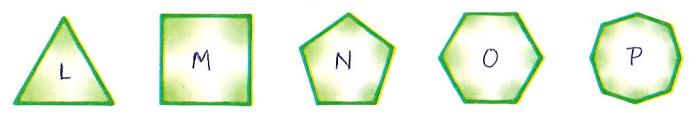4. Regular Polygons

A polygon with equal sides and angles is called regular.

 The polygon L is... The polygon M is... The polygon N is... The polygon O is... The polygon P is...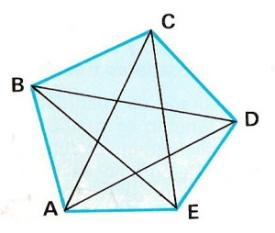5. Polygon parts

Look at the polygon ABCDE! Each point is a vertex. In the picture, there are 5 vertices: A, B, C, D and C. The sides are the line segments that make up the polygon. In this case, we have 5 sides: AB, BC, CD, DE and EA.

The diagonal is all line connecting two non consecutive vertices. In the picture, we can see that there are two diagonals per vertex. There are 5 diagonals: AC, AD, BD, BE and CE.

Look at the picture and answer:

 The A is... The AB is... The AC is... The AD is... The D is... The AE is... The B is... The C is... The CD is... The BE is... The BC is... The CA is...

 .adslot_S1 { display:inline-block; width: 336px; height: 280px; } @media (max-device-width:970px) { .adslot_S1 { display: none; } } If you are registered, please enter your data:   Name:  Password:   | Educational applications | Mathematics | In Spanish  | To print

«Arturo Ramo GarcĒa.-Record of intellectual property of Teruel (Spain) No 141, of 29-IX-1999
Plaza Playa de Aro, 3, 1║ DO 44002-TERUEL